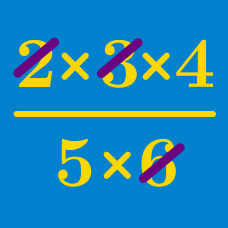Algebra

# Distributive Property

Evaluate $10 \times \left( \dfrac{1}{2} - \dfrac{3}{5} \right).$

It is given that

$3(8x + 9 ) + 5x = Nx + M$

is an algebraic identity. What is the value of $N$?

What is the sum of all the coefficients in the expansion of $2x(5x^3+6x+15)?$

What is the sum of all the coefficients in the expansion of the following expression: $3x(6y(x+3)+3x(y+4))?$

Simplify the expression

$8x + 3y + 2x + 10y.$

×

Problem Loading...

Note Loading...

Set Loading...